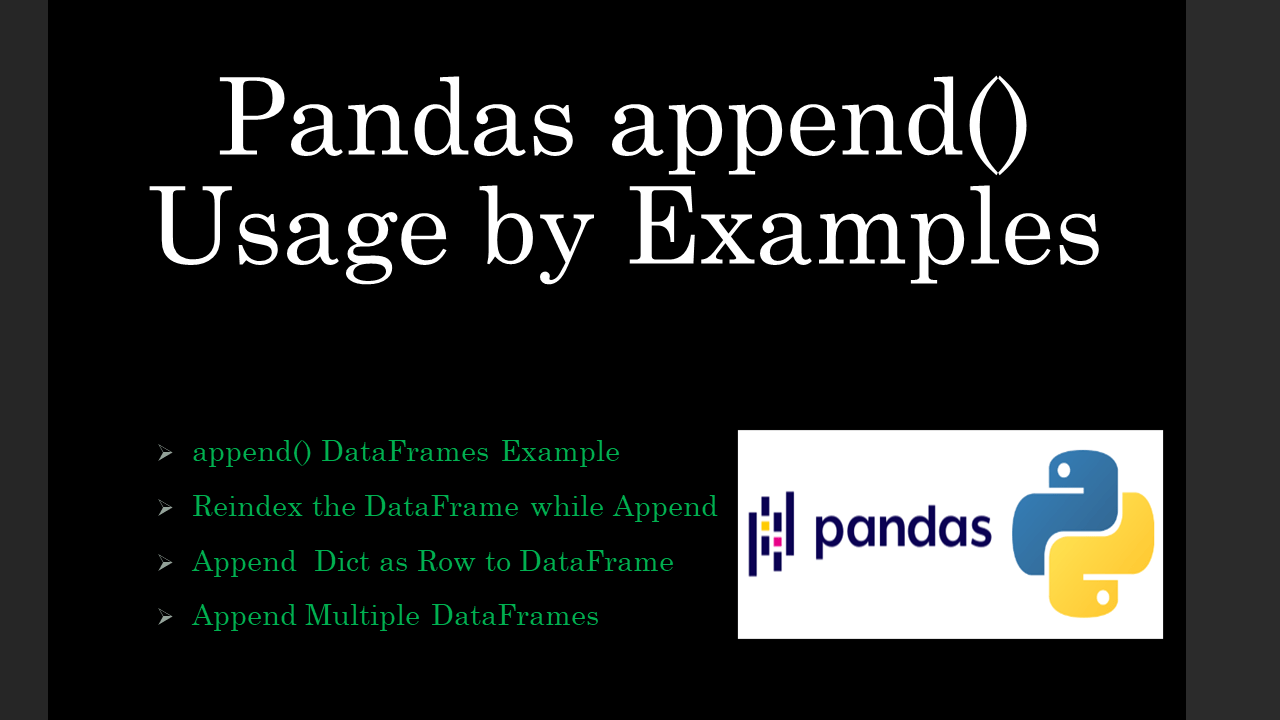# Pandas append() Usage by Examples

• Post author:
• Post category:Pandas

pandas.DataFrame.append() method is used to append one DataFrame row(s) and column(s) with another, it can also be used to append multiple (three or more) DataFrames. This method takes `other` (DataFrame you wanted to append), `ignore_index`, `verify_integrity`, `sort` as parameters and returns a new DataFrame with the combined result.

In this article, I will explain how to append pandas DataFrames with examples like appending rows, and columns, ignoring the index while appending, and more by using its parameters.

## 1. pandas append() Syntax

Below is the syntax of pandas.DataFrame.append() method.

``````
# Syntax of append()
DataFrame.append(other, ignore_index=False, verify_integrity=False, sort=False)
``````
• `other` – DataFrame or Series/dict-like object, or list of these.
• `ignore_index` – bool, default False. When set to True, It creates an axis with an incremental numeric number.
• `verify_integrity` – bool, default False. When set to True, raises an error for the duplicate index.
• `sort` – bool, default False.

Alternatively, you can also use pandas.DataFrame.concat() to concatenate DataFrames which can also be used to append.

## 2. Append Two DataFrames With the Same Columns

Let’s create a pandas DataFrame from Dict to explore this with an example.

``````
# Create two DataFrames with same columns
import pandas as pd

df1 = pd.DataFrame({'Courses': ["Spark","PySpark","Python","pandas"],
'Fee' : [20000,25000,22000,24000]})
print("First DataFrame:\n", df1)

'Fee': [25000,25200,24500,24900],
'Duration': ['30days','35days','40days','45days']})
print("Second DataFrame:\n", df2)
``````

Yields below output.

To append two DataFrames with the same columns in Padas, you can use the `concat()` function. It appends the column with NaN on the result for rows where the same column does not exist.

``````
# Append two DataFrames of same columns
# using append() function
df3 = df1.append(df2)
print("After appending DataFrames:\n", df3)
``````

Using this method you can also append list as a row to the DataFrame.

## 3. Pandas Append Two DataFrames Ignore Index

Append by default merges all rows including indices. if you want to ignore the index on DataFrames, you can set the new index on the pandas DataFrame by using `ignore_index=True` param.

``````
# Using append() with ignore_index
df2 = df.append(df1, ignore_index=True)
print(df2)
``````

Yields below output.

``````
# Output:
Courses    Fee Duration
0     Spark  20000      NaN
1   PySpark  25000      NaN
2    Python  22000      NaN
3    pandas  24000      NaN
4    Pandas  25000   30days
6  Hyperion  24500   40days
7      Java  24900   45days
``````

## 4. Append Dict as Row to DataFrame

Sometimes you would be required to append a dict as a row to DataFrame. The below example demonstrates how to do this with example. First, create a Dict and add it to the `df` object.

``````
# Append Dict as row to DataFrame
new_row = {'Courses':'Hyperion', 'Fee':24000}
df2=df.append(new_row, ignore_index=True)
print(df2)
``````

Yields below output.

``````
# Output:
Courses    Fee
0     Spark  20000
1   PySpark  25000
2    Python  22000
3    pandas  24000
4  Hyperion  24000
``````

## 5. Append Multiple DataFrames

To append multiple pandas DataFrames pass the DataFrames you wanted to append as a list to the append() method. Use `ingore_index=True` param to reset the index on pandas DataFrame to start from zero.

``````
# Create third DataFrame
df2 = pd.DataFrame({'Courses':['PHP','GO'],
'Duration':['30day','40days'],
'Fee':[10000,23000]})

# Appending multiple DataFrame
df3 = df.append([df1, df2], ignore_index=True)
print(df3)
``````

Yields below output

``````
# Output:
Courses    Fee Duration
Courses    Fee Duration
0     Spark  20000      NaN
1   PySpark  25000      NaN
2    Python  22000      NaN
3    pandas  24000      NaN
4    Pandas  25000   30days
6  Hyperion  24500   40days
7      Java  24900   45days
8       PHP  10000    30day
9        GO  23000   40days
``````

## 6. Complete Example of pandas append()

``````
# Example of pandas append()
import pandas as pd

df = pd.DataFrame({'Courses': ["Spark","PySpark","Python","pandas"],
'Fee' : [20000,25000,22000,24000]})

'Fee': [25000,25200,24500,24900],
'Duration': ['30days','35days','40days','45days']})

# Using append() method
df2 = df.append(df1)
print(df2)

# Using append() with ignore_index
df2 = df.append(df1, ignore_index=True)
print(df2)

# Create third DataFrame
df2 = pd.DataFrame({'Courses':['PHP','GO'],
'Duration':['30day','40days'],
'Fee':[10000,23000]})

# Appending multiple DataFrame
df3 = df.append([df1, df2], ignore_index=True)
print(df3)

# Append Dict as row to DataFrame
new_row = {'Courses':'Hyperion', 'Fee':24000}
df2=df.append(new_row, ignore_index=True)
print(df2)
``````

## Conclusion

By using the append() method you can append one DataFrame with another by rows and columns. This method takes other (pass list for multiple dataframes), ignore_index, verify_integrity, sort as parameters, and returns a new DataFrame with the combined result. Note that when you have an additional column on any of the DataFrame, it appends the column with NaN on the result for rows the same column does not exist.

## References

### Naveen

I am a Data Engineer with 20+ years of experience in transforming data into actionable insights. Over the years, I have honed my expertise in designing, implementing, and maintaining data pipelines with frameworks like Apache Spark, PySpark, Pandas, R, Hive and Machine Learning. My journey in the field of data engineering has been a continuous learning, innovation, and a strong commitment to data integrity. I have started this SparkByExamples.com to share my experiences with the data as I come across. You can learn more about me at LinkedIn